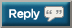# Thread: Let's Squat 5,000,000 pounds in 2021!

1. The past month:

July 22 front squats: 4230
July 24 low bar: 8740
July 29 front squats: 3755
August 3 low bar: 5245
August 9 front squats: 5870
August 11 low bar: 16,485
August 16 front squats: 4820
August 18 low bar: 15,140 (might have been 15,640 but lost count)

Total: 64,285
2,312,292 + 64,285 = 2,376,5772. recorded from 2 months out of the year... rest was bs and not written down.

2,487,142

i totaled 110,565 lbs to add to this3. recorded from 2 months out of the year... rest was bs and not written down.

2,487,142

i totaled 110,565 lbs to add to this
Last two weeks' work:

HIGH BAR
8 x 20kg = 160kg
5 x 40kg = 200kg
3 x 60kg = 180kg
1 x 77.5kg
3 x 77.5kg
3 x 77.5kg
3 x 77.5kg
3 x 77.5kg = 1007.5kg
= 1547.5kg

Skipped front squats due to knee pain

HIGH BAR
8 x 20kg = 160kg
5 x 40kg = 200kg
5 x 65kg
5 x 65kg = 650kg
1010kg

FRONT SQUAT
8 x 20kg = 160kg
4 x 40kg = 160kg
5 x 60kg
5 x 60kg = 600kg
920kg

3477.5kg (7667lb)

7667 + 2,487,142 = 2,494,8094. Last two weeks' work:

HIGH BAR
8 x 20kg = 160kg
5 x 40kg = 200kg
3 x 60kg = 180kg
1 x 77.5kg
3 x 77.5kg
3 x 77.5kg
3 x 77.5kg
3 x 77.5kg = 1007.5kg
= 1547.5kg

Skipped front squats due to knee pain

HIGH BAR
8 x 20kg = 160kg
5 x 40kg = 200kg
5 x 65kg
5 x 65kg = 650kg
1010kg

FRONT SQUAT
8 x 20kg = 160kg
4 x 40kg = 160kg
5 x 60kg
5 x 60kg = 600kg
920kg

3477.5kg (7667lb)

7667 + 2,487,142 = 2,494,809
+ 10,270 = 2,505,0795. Past 2 sessions

+ 16,141 = 2,521,2206. + 6,575 = 2,527,795

3 x 5 at 315 felt good being doable from the start after such a long break with the squat.7. + 11,835 = 2,539,630

Post-warmup:
4 x 8 at 2758. First contribution for a while.

HIGH BAR
8 x 20kg = 160kg
5 x 40kg = 200kg
3 x 65kg
8 x 65kg
8 x 65kg
8 x 65kg
8 x 65kg = 2275kg
Total = 2635kg

FRONT SQUAT
8 x 20kg = 160kg
4 x 40kg = 160kg
2 x 60kg
8 x 60kg
8 x 60kg
8 x 60kg = 1560kg
Total = 1880kg

HIGH BAR
8 x 20kg = 160kg
5 x 40kg = 200kg
3 x 60kg = 180kg
1 x 67.5kg
7 x 67.5kg
7 x 67.5kg
7 x 67.5kg
6 x 67.5kg = 1890kg
Total = 2430kg

FRONT SQUAT
8 x 20kg = 160kg
4 x 40kg = 160kg
2 x 62.5kg
7 x 62.5kg
7 x 62.5kg
7 x 62.5kg = 1437.5kg
Total = 1757.5kg

8702.5kg = 19189lb

19189 + 2,539,630 = 2,558,8199. High Bar
10 x 20kg = 200kg
5 x 40kg = 200kg
3 x 60kg
5 x 60kg
5 x 60kg = 780kg
Total: 1180kg

Front Squat
8 x 20kg = 160kg
4 x 40kg = 160kg
5 x 55kg
5 x 55kg = 550kg
Total: 870kg

High Bar
10 x 20kg = 200kg
5 x 40kg = 200kg
3 x 60kg
9 x 60kg
8 x 60kg
7 x 60kg = 1620kg
Total: 2020kg

Front Squat
8 x 20kg = 160kg
4 x 40kg = 160kg
2 x 55kg
8 x 55kg
6 x 55kg
5 x 55kg = 1155kg
Total: 1475kg

5545kg = 12,226lb

12,226 + 2,558,819 = 2,571,04510. 2,571,045 + 7,160 = 2,578,205

Working sets:
3 x 5 at 27511. Since I've been an active member, ECGordyn was like this forum's conscience for the squat... hope you check in soon OP. Feels weird quoting myself for the next installment on this thread honestly.

2,571,045 + 7,160 = 2,578,205

Working sets:
3 x 5 at 275
From yesterday...

Warm up: 2,360
Working sets: 9,000

2,578,205 + 11,360 = 2,589,565

(4 x 10 at 225)12. High Bar
10 x 20kg = 200kg
5 x 40kg = 200kg
3 x 62.5kg
9 x 62.5kg
8 x 62.5kg
7 x 62.5kg
6 x 62.5kg = 2062.5kg
Total: 2462.5kg

Front Squat
8 x 20kg = 160kg
4 x 40kg = 160kg
2 x 55kg
9 x 55kg
7 x 55kg
6 x 55kg
5 x 55kg = 1595
Total: 1915kg

High Bar
10 x 20kg = 200kg
5 x 40kg = 200kg
3 x 65kg
9 x 65kg
8 x 65kg
7 x 65kg
6 x 65kg
5 x 65kg = 2470kg
Total: 2870kg

Front Squat
8 x 20kg = 160kg
4 x 40kg = 160kg
2 x 57.5kg
9 x 57.5kg
7 x 57.5kg
6 x 57.5kg
5 x 57.5kg
5 x 57.5kg = 1955kg
Total: 2275kg

9522.5kg = 20,997lb

20,997 + 2,589,565 = 2,610,56213. 2,610,562 + 15,860 = 2,626,422

Warm up: 2,360
Working sets: 13,500

6 x 10 at 22514. How am I double posting this thread again?

2,626,422 + 5,405 = 2,631,827

Warm up: 2,570
Working weight: 2,835

3 x 3 at 31515. I'm on a roll here lol.

2,631,827 + 10,270 = 2,642,097

warm ups: 3,310

working sets: 6,960

4 x 6 at 290

Might have miscounted a set of warmups as this was from yesterday, but I think it's accurate.#### Posting Permissions

• You may not post new threads
• You may not post replies
• You may not post attachments
• You may not edit your posts